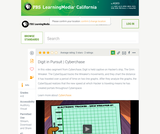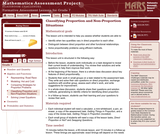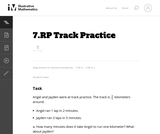Updating search results...

# 23 Results

View
Selected filters:
• WY.Math.7.RP.A.2dOnly Sharing Permitted
CC BY-NC-ND
Rating
5.0 stars

This lesson unit is intended to help you assess whether students recognize relationships of direct proportion and how well they solve problems that involve proportional reasoning. In particular, it is intended to help you identify those students who: use inappropriate additive strategies in scaling problems, which have a multiplicative structure; rely on piecemeal and inefficient strategies such as doubling, halving, and decomposition, and have not developed a single multiplier strategy for solving proportionality problems; and see multiplication as making numbers bigger, and division as making numbers smaller.

Subject:
Numbers and Operations
Material Type:
Assessment
Lesson Plan
Provider:
Shell Center for Mathematical Education
Provider Set:
Mathematics Assessment Project (MAP)
04/26/2013Educational Use
Rating
0.0 stars

The CyberSquad tracks Digital position in time and then studies graphs to figure out what Hacker is scheming in this video from Cyberchase.

Subject:
Mathematics
Physics
Material Type:
Lecture
Provider:
PBS LearningMedia
Provider Set:
PBS Learning Media: Multimedia Resources for the Classroom and Professional Development
Author:
U.S. Department of Education
WNET
09/25/2008Only Sharing Permitted
CC BY-NC-ND
Rating
4.5 stars

This lesson unit is intended to help assess how well students are able to interpret and use scale drawings to plan a garden layout. This involves using proportional reasoning and metric units.

Subject:
Algebra
Geometry
Ratios and Proportions
Material Type:
Assessment
Lesson Plan
Provider:
Shell Center for Mathematical Education
Provider Set:
Mathematics Assessment Project (MAP)
04/26/2013Only Sharing Permitted
CC BY-NC-ND
Rating
4.0 stars

This lesson unit is intended to help you assess how well students are able to: solve simple problems involving ratio and direct proportion; choose an appropriate sampling method; and collect discrete data and record them using a frequency table.

Subject:
Education
Geometry
Measurement and Data
Ratios and Proportions
Material Type:
Assessment
Lecture Notes
Lesson Plan
Teaching/Learning Strategy
Provider:
Shell Center for Mathematical Education
Provider Set:
Mathematics Assessment Project (MAP)
Author:
http://map.mathshell.org/
04/26/2013Educational Use
Rating
0.0 stars

The CyberSquad tests which broom can travel the furthest in five seconds in this video from Cyberchase.

Subject:
Mathematics
Physics
Material Type:
Lecture
Provider:
PBS LearningMedia
Provider Set:
PBS Learning Media: Multimedia Resources for the Classroom and Professional Development
Author:
U.S. Department of Education
WNET
07/08/2008Educational Use
Rating
0.0 stars

In this lesson designed to enhance literacy skills, students learn how to read and interpret a distance&ndash;time graph.

Subject:
Mathematics
Algebra
Physics
Material Type:
Activity/Lab
Provider:
PBS LearningMedia
Provider Set:
PBS Learning Media Common Core Collection
Author:
Walmart Foundation
WGBH Educational Foundation
08/21/2012Only Sharing Permitted
CC BY-NC-ND
Rating
4.0 stars

This lesson unit is intended to help teachers assess how well students are able to interpret percent increase and decrease, and in particular, to identify and help students who have the following difficulties: translating between percents, decimals, and fractions; representing percent increase and decrease as multiplication; and recognizing the relationship between increases and decreases.

Subject:
Ratios and Proportions
Material Type:
Assessment
Lesson Plan
Provider:
Shell Center for Mathematical Education
Provider Set:
Mathematics Assessment Project (MAP)
04/26/2013Conditional Remix & Share Permitted
CC BY-NC
Rating
0.0 stars

Four full-year digital course, built from the ground up and fully-aligned to the Common Core State Standards, for 7th grade Mathematics. Created using research-based approaches to teaching and learning, the Open Access Common Core Course for Mathematics is designed with student-centered learning in mind, including activities for students to develop valuable 21st century skills and academic mindset.

Subject:
Mathematics
Material Type:
Full Course
Provider:
Pearson
10/06/2016Rating
0.0 stars

Proportional Relationships

Type of Unit: Concept

Prior Knowledge

Students should be able to:

Understand what a rate and ratio are.
Make a ratio table.
Make a graph using values from a ratio table.

Lesson Flow

Students start the unit by predicting what will happen in certain situations. They intuitively discover they can predict the situations that are proportional and might have a hard time predicting the ones that are not. In Lessons 2–4, students use the same three situations to explore proportional relationships. Two of the relationships are proportional and one is not. They look at these situations in tables, equations, and graphs. After Lesson 4, students realize a proportional relationship is represented on a graph as a straight line that passes through the origin. In Lesson 5, they look at straight lines that do not represent a proportional relationship. Lesson 6 focuses on the idea of how a proportion that they solved in sixth grade relates to a proportional relationship. They follow that by looking at rates expressed as fractions, finding the unit rate (the constant of proportionality), and then using the constant of proportionality to solve a problem. In Lesson 8, students fine-tune their definition of proportional relationship by looking at situations and determining if they represent proportional relationships and justifying their reasoning. They then apply what they have learned to a situation about flags and stars and extend that thinking to comparing two prices—examining the equations and the graphs. The Putting It Together lesson has them solve two problems and then critique other student work.

Gallery 1 provides students with additional proportional relationship problems.

The second part of the unit works with percents. First, percents are tied to proportional relationships, and then students examine percent situations as formulas, graphs, and tables. They then move to a new context—salary increase—and see the similarities with sales taxes. Next, students explore percent decrease, and then they analyze inaccurate statements involving percents, explaining why the statements are incorrect. Students end this sequence of lessons with a formative assessment that focuses on percent increase and percent decrease and ties it to decimals.

Students have ample opportunities to check, deepen, and apply their understanding of proportional relationships, including percents, with the selection of problems in Gallery 2.

Subject:
Mathematics
Ratios and Proportions
Material Type:
Unit of Study
Provider:
PearsonConditional Remix & Share Permitted
CC BY-NC
Rating
0.0 stars

Students analyze the graph of a proportional relationship in order to find the approximate constant of proportionality, to write the related formula, and to create a table of values that lie on the graph.Key ConceptsThe constant of proportionality determines the steepness of the straight-line graph that represents a proportional relationship. The steeper the line is, the greater the constant of proportionality.On the graph of a proportional relationship, the constant of proportionality is the constant ratio of y to x, or the slope of the line.A proportional relationship can be represented in different ways: a ratio table, a graph of a straight line through the origin, or an equation of the form y = kx, where k is the constant of proportionality.Goals and Learning ObjectivesIdentify the constant of proportionality from a graph that represents a proportional relationship.Write a formula for a graph that represents a proportional relationship.Make a table for a graph that represents a proportional relationship.Relate the constant of proportionality to the steepness of a graph that represents a proportional relationship (i.e., the steeper the line is, the greater the constant of proportionality).

Subject:
Ratios and Proportions
Material Type:
Lesson Plan
Provider:
Pearson
09/21/2015Conditional Remix & Share Permitted
CC BY-NC
Rating
0.0 stars

Students connect percent to proportional relationships in the context of sales tax.Key ConceptsWhen there is a constant tax percent, the total cost for items purchase—including the price and the tax—is proportional to the price.To find the cost, c , multiply the price of the item, p, by (1 + t), where t is the tax percent, written as a decimal: c = p(1 + t).The constant of proportionality is (1 + t) because of the structure of the situation:c = p + tp = p(1 + t).Because of the distributive property, multiplying the price by (1 + t) means multiplying the price by 1, then multiplying the price by t, and then taking the sum of these products.Goals and Learning ObjectivesFind the total cost in a sales tax situation.Understand that a proportional relationship only exists between the price of an item and the total cost of the item if the sales tax is constant.Find the constant of proportionality in a sales tax situation.Make a graph of an equation showing the relationship between the price of an item and the total amount paid.

Subject:
Ratios and Proportions
Material Type:
Lesson Plan
Provider:
Pearson
09/21/2015Conditional Remix & Share Permitted
CC BY-NC
Rating
0.0 stars

Students create equations, tables, and graphs to show the proportional relationships in sales tax situations.Key ConceptsThe quantities—price, tax, and total cost—can each be known or unknown in a given situation, but if you know two quantities, you can figure out the missing quantity using the structure of the relationship among them.If either the price or the total cost are unknown, you can write an equation of the form y = kx, with k as the known value (1 + tax), and solve for x or y.If the tax is the unknown value, you can write an equation of the form y = kx and solve for k, and then subtract 1 from this value to find the tax (as a decimal value).Building a general model for the relationship among all three quantities helps you sort out what you know and what you need to find out.Goals and Learning ObjectivesMake a table to organize known and unknown quantities in a sales tax problem.Write and solve an equation to find an unknown quantity in a sales tax problem.Make a graph to represent a table of values.Determine the unknown amount—either the price of an item, the amount of the sales tax, or the total cost—in a sales tax situation when given the other two amounts.

Subject:
Ratios and Proportions
Material Type:
Lesson Plan
Provider:
Pearson
09/21/2015Conditional Remix & Share Permitted
CC BY-NC
Rating
0.0 stars

Students look at the relationship between the number of flags manufactured and the stars on the flag and determine whether it represents a proportional relationship.Key ConceptsThe form of the equation of a proportional relation is y = kx, where k is the constant of proportionality.A graph of a proportional relationship is a straight line that passes through the origin.The constant of a proportionality in a graph of a proportional relationship is the constant ratio of y to x (the slope of the line).Goals and Learning ObjectivesIdentify the constant of proportionality in a proportional relationship based on a real-world problem situation.Write a formula using the constant of proportionality.Analyze a graph of a proportional relationship.Make a graph and determine if it represents a proportional relationship.Identify the constant of proportionality in a graph of a proportional relationship.

Subject:
Ratios and Proportions
Material Type:
Lesson Plan
Provider:
Pearson
09/21/2015Conditional Remix & Share Permitted
CC BY-NC
Rating
0.0 stars

Students represent and solve percent decrease problems.Key ConceptsWhen there is a percent decrease between a starting amount and a final amount, the relationship can be represented by an equation of the form y = kx where y is the final amount, x is the starting amount, and k is the constant of proportionality, which is equal to 1 minus the percent change, p, represented as a decimal: k = 1 – p, so y = (1 – p)x.The constant of proportionality k has the value it does—a number less than 1—because of the way the distributive property can be used to simplify the expression for the starting amount decreased by a percent of the starting amount: x – x(p) = x(1 – p).Goals and Learning ObjectivesDetermine the unknown amount—either the starting amount, the percent change, or the final amount—in a percent decrease situation when given the other two amounts.Make a table to represent a percent decrease problem.Write and solve an equation to represent a percent decrease problem.

Subject:
Ratios and Proportions
Material Type:
Lesson Plan
Provider:
Pearson
09/21/2015Conditional Remix & Share Permitted
CC BY-NC
Rating
0.0 stars

Students represent and solve percent increase problems.Key ConceptsWhen there is a percent increase between a starting amount and a final amount, the relationship can be represented by an equation of the form y = kx where y is the final amount, x is the starting amount, and k is the constant of proportionality, which is equal to 1 plus the percent change, p, represented as a decimal: k = 1 + p, so y = (1 + p)x.The constant of proportionality k has the value it does—a number greater than 1—because of the way the distributive property can be used to simplify the expression for the starting amount increased by a percent of the starting amount: x + x(p) = x(1 + p).Goals and Learning ObjectivesDetermine the unknown amount—either the starting amount, the percent change, or the final amount—in a percent increase situation when given the other two amounts.Make a table to represent a percent increase problem.Write and solve an equation to represent a percent increase problem.

Subject:
Ratios and Proportions
Material Type:
Lesson Plan
Provider:
Pearson
09/21/2015Conditional Remix & Share Permitted
CC BY-NC
Rating
0.0 stars

Students have an opportunity to review their own work on the Self Check in the previous lesson, consider feedback that addresses specific aspects of their work, examine a different approach to the problem from the Self Check, and then use what they learned to solve a closely related problem.Key ConceptsStudents reflect on their work, review and critique student work on the same problem, and then apply their learning to solve a similar problem.Goals and Learning ObjectivesUse teacher comments to refine their solution strategies for a proportional relationship problemDeepen their understanding of proportional relationships.Synthesize and connect strategies for representing and investigating proportional relationships.Critique given student work involving proportional relationships.Apply deepened understanding of proportional relationships to a new problem situation.

Subject:
Mathematics
Material Type:
Lesson Plan
Provider:
Pearson
09/21/2015Only Sharing Permitted
CC BY-NC-ND
Rating
5.0 stars

This lesson unit is intended to help teachers assess whether students are able to: identify when two quantities vary in direct proportion to each other; distinguish between direct proportion and other functional relationships; and solve proportionality problems using efficient methods.

Subject:
Ratios and Proportions
Material Type:
Assessment
Lesson Plan
Provider:
Shell Center for Mathematical Education
Provider Set:
Mathematics Assessment Project (MAP)
04/26/2013Educational Use
Rating
0.0 stars

In this video from Cyberchase, the CyberSquad figures out how fast their broom must go so they can reach Motherboard before Wicked.

Subject:
Mathematics
Physics
Material Type:
Lecture
Provider:
PBS LearningMedia
Provider Set:
PBS Learning Media: Multimedia Resources for the Classroom and Professional Development
Author:
U.S. Department of Education
WNET
07/09/2008Unrestricted Use
CC BY
Rating
0.0 stars

The purpose of this task is to engage students in Standard for Mathematical Practice 4, Model with mathematics and as such, the question as it is worded cannot be answered without making some assumptions. For example, if the items that are purchased do not have the same value, then the price reduction depends on the cost of the items.

Subject:
Mathematics
Material Type:
Activity/Lab
Provider:
Illustrative Mathematics
Provider Set:
Illustrative Mathematics
Author:
Illustrative Mathematics
05/01/2012Unrestricted Use
CC BY
Rating
0.0 stars

This problem can be solved in more than one way. The choice in solution method may reflect the comfort and mathematical sophistication of the student.

Subject:
Mathematics
Material Type:
Activity/Lab
Provider:
Illustrative Mathematics
Provider Set:
Illustrative Mathematics
Author:
Illustrative Mathematics
05/01/2012Unrestricted Use
CC BY
Rating
0.0 stars

This is a multi-step problem since it requires more than two steps no matter how it is solved. The problem is not scaffolded for the student, but each step is straightforward and should follow from the previous with a careful reading of the problem.

Subject:
Mathematics
Material Type:
Activity/Lab
Provider:
Illustrative Mathematics
Provider Set:
Illustrative Mathematics
Author:
Illustrative Mathematics
05/01/2012Conditional Remix & Share Permitted
CC BY-NC-SA
Rating
0.0 stars

Parts (a) and (b) of the task ask students to find the unit rates that one can compute in this context. Part (b) does not specify whether the units should be laps or km, so answers can be expressed using either one.

Subject:
Mathematics
Material Type:
Activity/Lab
Provider:
Illustrative Mathematics
Provider Set:
Illustrative Mathematics
Author:
Illustrative Mathematics
05/01/2012Educational Use
Rating
0.0 stars

In this lesson designed to enhance literacy skills, students examine how to use fractions to measure and help conserve freshwater resources.

Subject:
Mathematics
Material Type:
Activity/Lab
Provider:
PBS LearningMedia
Provider Set:
PBS Learning Media Common Core Collection
Author:
Walmart Foundation
WGBH Educational Foundation